# What is a TVM calculation

 The Time-Value-Money-SolverFinancial mathematics with electronic tools

Mathematical content:

Solutions to problems in the field of financial mathematics
Brief summary:
With the TVM-Solver, the users of the TI-83 / TI-83 + already had a convenient tool for solving financial mathematical tasks.
Since August 2000 there is also a Flash application of this TVM solver for the TI-89 / TI-92 + as well as a version for Excel. This greatly reduces the computational effort in the field of financial mathematics and very extensive application-oriented examples can also be discussed.
Curriculum reference:
3rd year HAK
Time required:
approx. 1 hour a week to introduce the solver
Media environment:
Excel or TI-89 or TI-92 Plus or TI-83 / TI-83 Plus.

Basics

With the help of the TVM solver you can easily calculate all variables that occur in the area of ​​compound interest and pension calculations. The solver works with decursive theoretical interest.
The formula according to which the calculations are made is (see also AMMU November 98; page 1):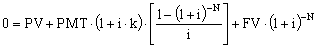With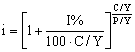.

Designations:

N .... Number of payment periods; Number of installments; (Duration in years)
I% ... Annual interest rate; nominal decursive annual interest rate
PV ... P.resent Value; Present value
PMT ... P.ayment amount; Guess
FV ... F.uture Value; F.inal Value; Future value; Final value
P / Y ... Number of payment periods per year; Payments (regularly) per year [PpY]
C / Y ... Number of compounding periods per year; Compound interest periods per year [CpY]
PMT ... END BEGIN
END: means payment at the end of a pension period (in arrears; k = 0)
BEGIN: means payment at the beginning of the pension period (in advance; k = 1)

All amounts that you receive will be entered positively. All amounts that you must pay are entered with a negative (-) sign.

The following examples are intended to give an overview of the possible uses of the TVM solver. Since the input into each of the solvers is very similar, the solution becomes
each task is only ever represented with a part of the solver.

Example 1: Compound interest calculation (TI-92 + / TI-89)
Ms. A. puts an amount of € 50,000 in a savings account.
What amount can she dispose of after 10 years with i = 4% (theoretical interest rate)?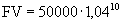N = 10 10 years I% = 4 nominal annual interest PV = -50000 note the negative sign PMT = 0 no regular installment payments FV =? searched P / Y = 1 input 1 (time in years) C / Y = 1 one compound interest period per year PMT: This entry is irrelevant for compound interest calculations To do this with the TI-89 / TI92 +, first press the button APPS ; choose 1: FlashApps... and then Finance.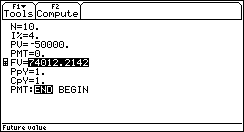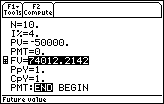Enter the values ​​ as described in the input menu of the TVM solver. Then place the cursor next to the quantity to be calculated and press the key F2 to solve the task. A marker appears next to the calculated size.
Answer: After 10 years, Ms. A. can dispose of a capital of € 74,012.21.

Example 2: Compound interest calculation (TI-83 + / TI-89)
a) What amount must Ms. A. put in a savings account today if she will have over in 15 years
Would like to dispose of € 100,000? (i4 = 1.5%, theoretical interest)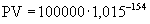N = 15 15 years I% = 6 nominal annual interest = 1.5 * 4 PV =? searched; note the negative sign! PMT = 0 no regular installment payments FV = 100000 wanted P / Y = 1 input 1 C / Y = 4 4 compound interest periods per year PMT: This entry is optional for compound interest calculations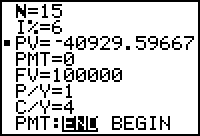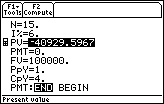Answer: Today Ms A. has to pay in € 40,929.60 in order to be able to dispose of € 100,000 in 15 years

 b) What would be the amount to be paid in at i12 = 0,5%?  I% = 6 nominal annual interest rate = 0.5 * 12 C / Y = 12 12 compound interest periods per year The amount to be deposited is € 40,748.24.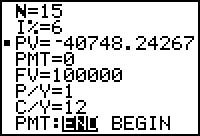Example 3: (Pension calculation; Excel, TI-89)
At the beginning of each month, Mr A. would like to withdraw € 2,000 from a savings account for a total of 10 years. What amount must be in the savings book immediately before the first payout if there should be no remainder in the savings book after the last monthly installment has been paid out?
i2=2%

 N = 120 120 monthly installments I% = 4 nominal annual interest = 2 * 2 PV =? searched; note the negative sign! PMT = 2000 monthly payout FV = 0 no remainder at the end of the term P / Y = 12 12 installments per year C / Y = 2 2 compound interest periods per year; Attention automatic! PMT: BEGIN; Payments at the beginning of the period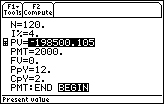To solve the problem with the TVM-Solver for Excel, enter the given values ​​in the table and confirm each entry with ENTER.
Use the mouse to click on the button with the size you want to calculate.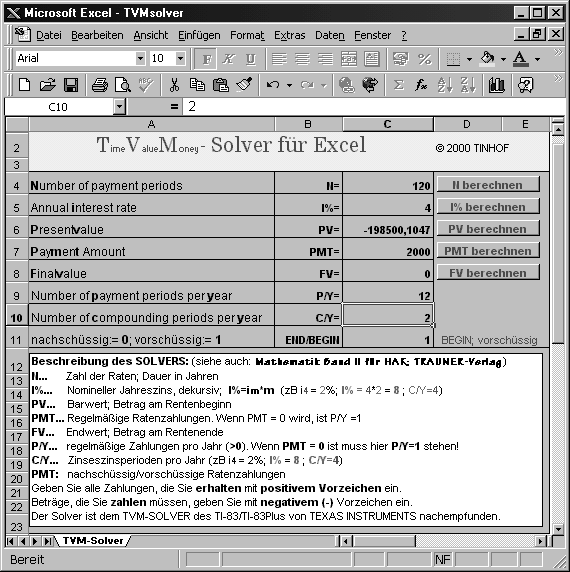Example 4: (Pension calculation, calculation of the interest rate: Excel; TI-83)
Mr. Max Muster receives a personal loan from his bank on the following terms:
Loan amount: € 100,000
Legal fee € 800
Processing fee € 1,000
Payout amount € 98 200, -
60 monthly installments of € 1947.52 for the first time 1 month after loan disbursement
What is the effective interest rate?

N = 60 60 monthly installments
I% =? Looking for nominal annual interest
PV = 98200 amount paid out
PMT = -1947.52 monthly rate; note the negative sign!
FV = 0 no remainder at the end of the term
P / Y = 12 12 installments per year
C / Y = 1 1 compound interest period per year
PMT: END; Payments at the end of the period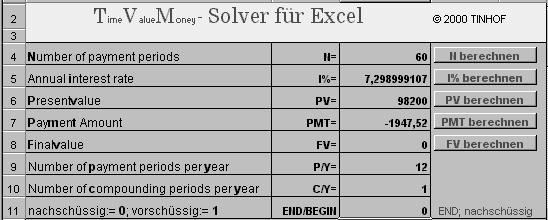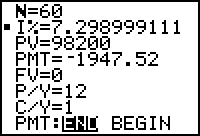Answer: The actual effective interest rate is 7.299%.

The effective interest rate given by the bank is slightly lower than the calculated effective interest rate.
What is the reason for that?
Answer: According to the BWG 1994, the bank does not take the legal fee into account when calculating the effective interest rate. This results in:
PV = 99,000 amount paid out 98,200 + 800 = 99,000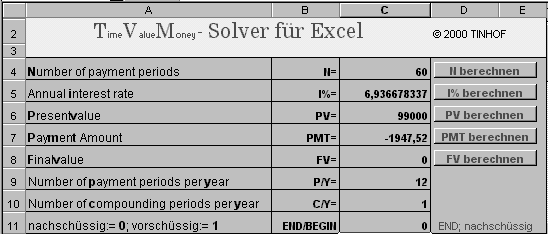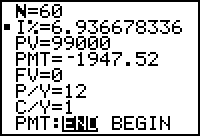The effective interest rate given by the bank is 6.9367%.

Example 5: (Pension conversion, duration of pension, remainder of pension)

Mr. M. is entitled to a quarterly pension of € 20,000 per installment starting on November 1, 2000, nine times in advance.
Instead of this quarterly pension, he wants a monthly pension with an installment of € 5,000.
The first payment of the monthly installment is to take place on May 1st, 2001.
How many full monthly installments can Mr M. draw and what amount (remainder of the pension) remains in the account on the day the last full monthly installment is paid out. i2 = 2,5%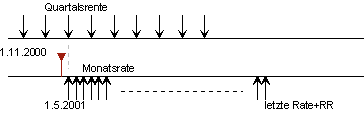Step 1: Calculate the present value of the quarterly pension. N = 9 9 quarterly rates I% = 5 nominal annual interest PV =? Cash value; note the negative sign! PMT = 20000 monthly installment; FV = 0 no remainder at the end of the term P / Y = 4 4 installments per year C / Y = 2 2 compound interest periods per year; Watch out for the automatic! PMT: BEGIN; advance installments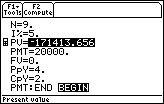Step 2: The calculated present value is five Months compounded. N = 5/12 5/12 YearsI% = 5 nominal annual interest PV = -171413.656 present value; note the negative sign! PMT = 0 no installment payment; FV = 174 977.406 Value of the quarterly pension on April 1, 2001 P / Y = 1 payments per yearC / Y = 2 2 compound interest periods per year; Note the automatic! PMT: any The value of the quarterly pension on April 1, 2001 is € 175,977.41 and is the present value of a follow up Monthly pension with first payment on 1.5.2001.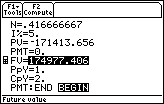Step 3: The term of the monthly pension is calculated from the present value. N =? wanted, number of monthly installments I% = 5 nominal annual interest PV = -174977.406 present value of monthly pension; negative PMT = 5000 installments; FV = 0 final value of the monthly pension P / Y = 12 payments per year C / Y = 2 2 compound interest periods per year; Note the automatic! PMT: END; additional pension 37 full monthly installments of € 5000 each are paid out. The remainder of the pension (on the day of the last full installment) is the final value of the topoor monthly pension.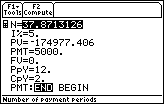Step 4: Calculation of the pension balance on the day of the last full installment. (Estimate: approx. 87% of 5000 = 4350, -) N = 37 sought, number of monthly installments I% = 5 nominal annual interest PV = -174977.406 present value of monthly pension; negative PMT = 5000 installments; FV =? searched; Pension remainder P / Y = 12 payments per year C / Y = 2 2 compound interest periods per year PMT: END; additional pension The remainder of the pension (at the same time as the last full installment) is € 4,339.82.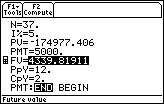What to look out for
Black box: The TVM solver is a classic tool that can be used in the classroom in the form of a black box. However, it is advisable to first use the "classical" method to calculate some elementary tasks, ie with the help of the sum formula for geometric sequences. The students need to understand the background of the calculation, only then does the TVM solver make sense.
The main aim of the solver is to reduce the computational effort involved in financial mathematical problems and to provide more precise results.
The teacher can concentrate on the problems of financial mathematics in the classroom and purely computational problems take a back seat. Financial mathematics can thus be made more attractive. (Teaching practice shows that even without a TVM solver, the pension formula will sooner or later be used as a black box by every student.)
Protocol: Since the mathematical solution of a financial mathematics problem is carried out almost entirely by the TVM solver, a suitable presentation of the solution to pay attention.
The keeping of a protocol that explains the individual calculation steps carried out in key words has proven to be very effective here. A list of the values ​​entered (input log) and a graphical representation of the payment flows in the form of a time line should supplement the description of the individual calculation steps.
English terms: The English technical terms are becoming more and more popular in the financial world. It therefore makes sense to work with the English names of the solver in mathematics lessons as well.

Where can you get the solvers from?

The TVM solver is already stored on the TI-83 / TI-83plus upon delivery.
You can find the solver for the TI-89 / TI-92plus as a free flash application on the Internet. To load these applications onto the computer, you need the TI-Graph-Link cable with the latest software.

http://www.ti.com/calc/flash/89.htm
http://www.ti.com/calc/flash/92p.htm
http://www.ti.com/calc/oesterreich/

The TVM solver for Excel was created by me, is equivalent to the TI TVM solver and is also available free of charge on the Internet. The internet addresses are: